# Презентация, доклад Currents in мetals

Вы можете изучить и скачать доклад-презентацию на тему Currents in мetals. Презентация на заданную тему содержит 36 слайдов. Для просмотра воспользуйтесь проигрывателем, если материал оказался полезным для Вас - поделитесь им с друзьями с помощью социальных кнопок и добавьте наш сайт презентаций в закладки!
Презентации» Физика» Currents in мetals

Слайды и текст этой презентации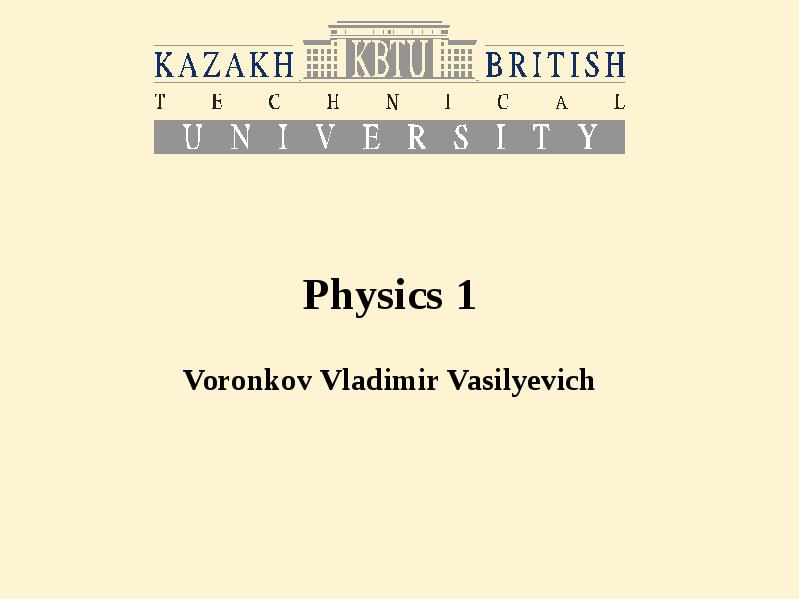Описание слайда: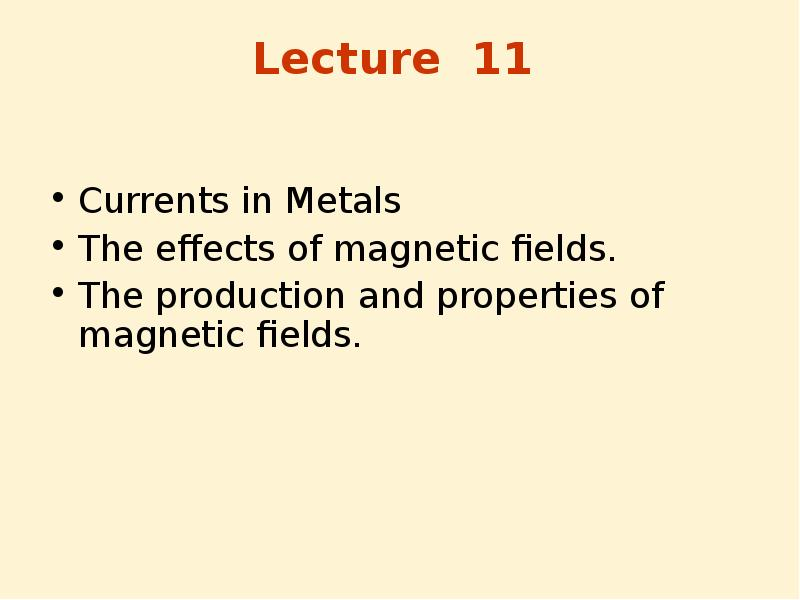Описание слайда:
Lecture 11 Currents in Metals The effects of magnetic fields. The production and properties of magnetic fields.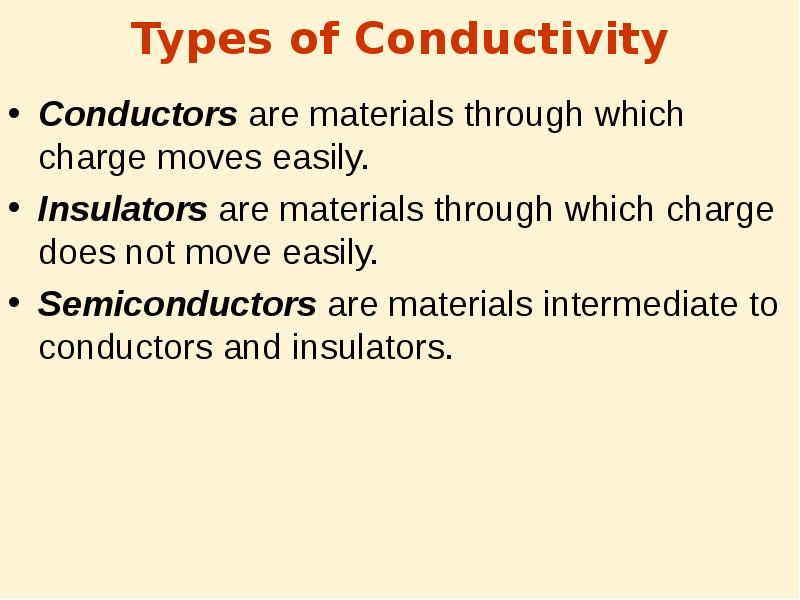Описание слайда:
Types of Conductivity Conductors are materials through which charge moves easily. Insulators are materials through which charge does not move easily. Semiconductors are materials intermediate to conductors and insulators.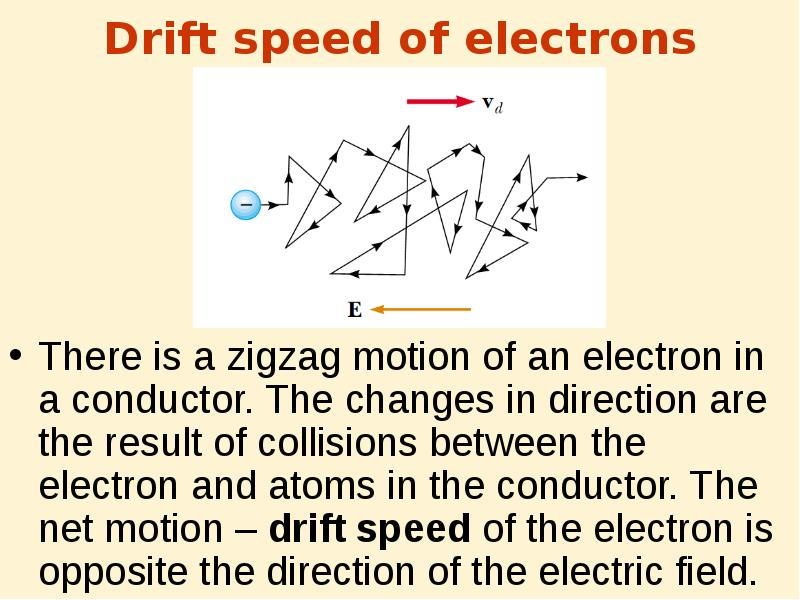Описание слайда:
Drift speed of electrons There is a zigzag motion of an electron in a conductor. The changes in direction are the result of collisions between the electron and atoms in the conductor. The net motion – drift speed of the electron is opposite the direction of the electric field.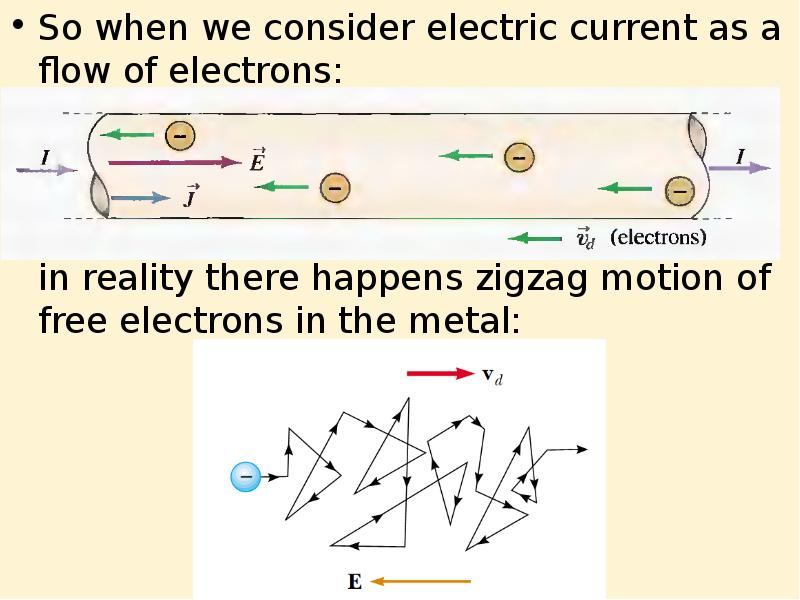Описание слайда:
So when we consider electric current as a flow of electrons: So when we consider electric current as a flow of electrons: in reality there happens zigzag motion of free electrons in the metal: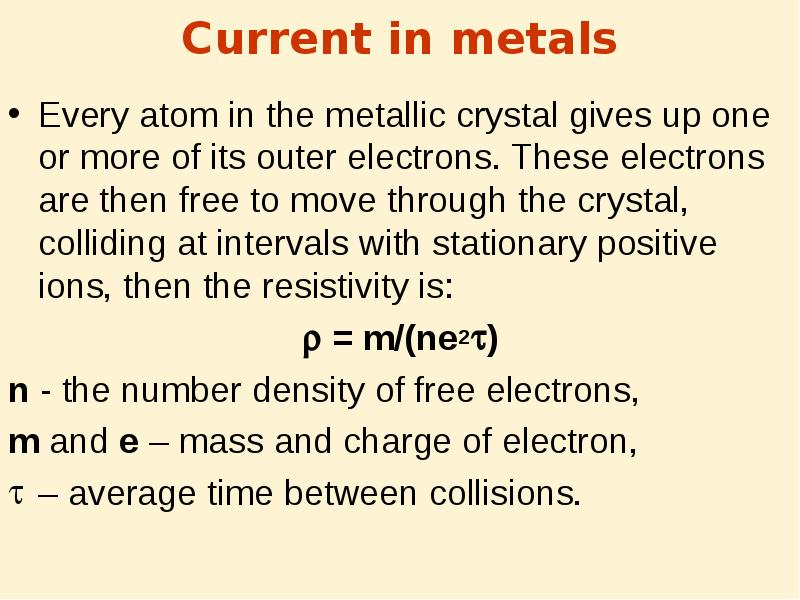Описание слайда:
Current in metals Every atom in the metallic crystal gives up one or more of its outer electrons. These electrons are then free to move through the crystal, colliding at intervals with stationary positive ions, then the resistivity is:  = m/(ne2) n - the number density of free electrons, m and e – mass and charge of electron, – average time between collisions.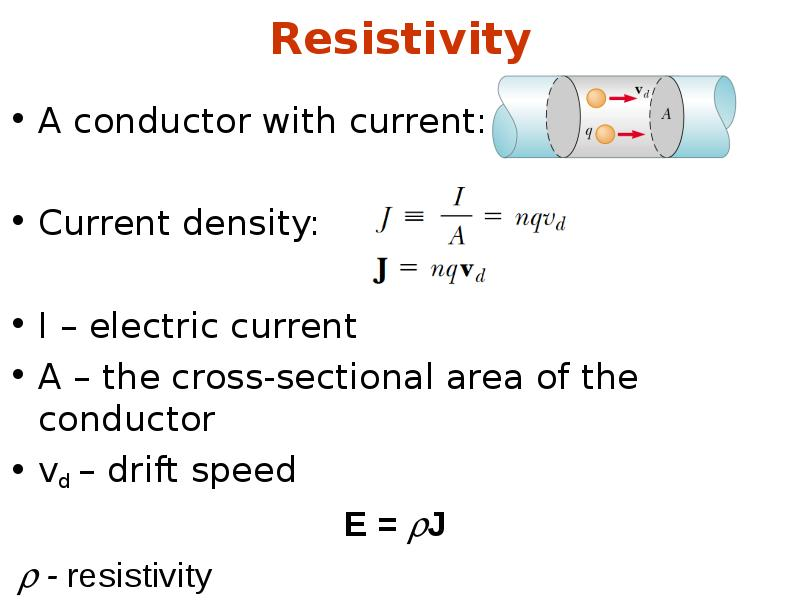Описание слайда:
Resistivity A conductor with current: Current density: I – electric current A – the cross-sectional area of the conductor vd – drift speed E = J - resistivity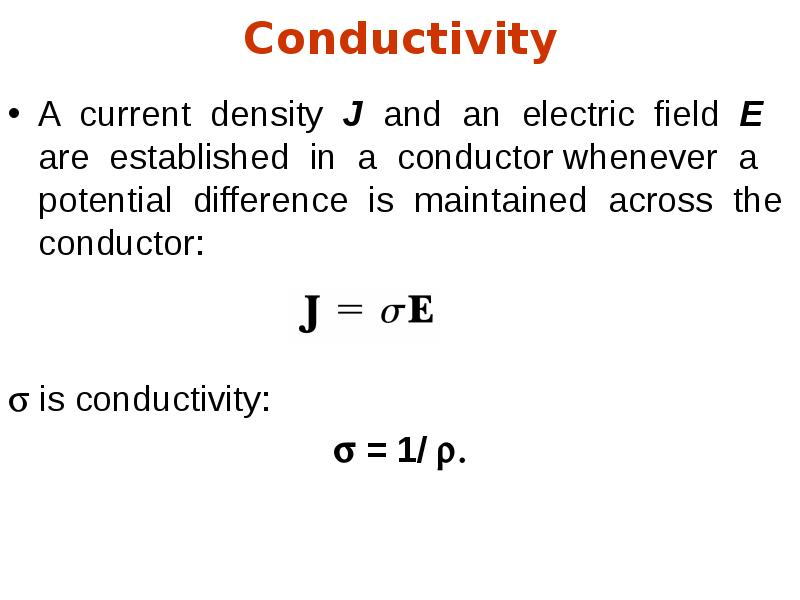Описание слайда:
Conductivity A current density J and an electric field E are established in a conductor whenever a potential difference is maintained across the conductor:  is conductivity:  = 1/ 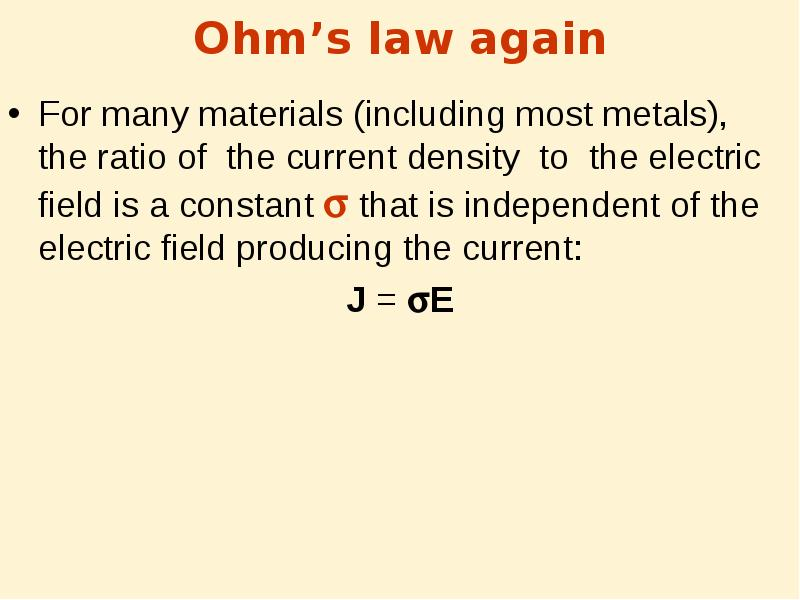Описание слайда:
Ohm’s law again For many materials (including most metals), the ratio of the current density to the electric field is a constant  that is independent of the electric field producing the current: J = E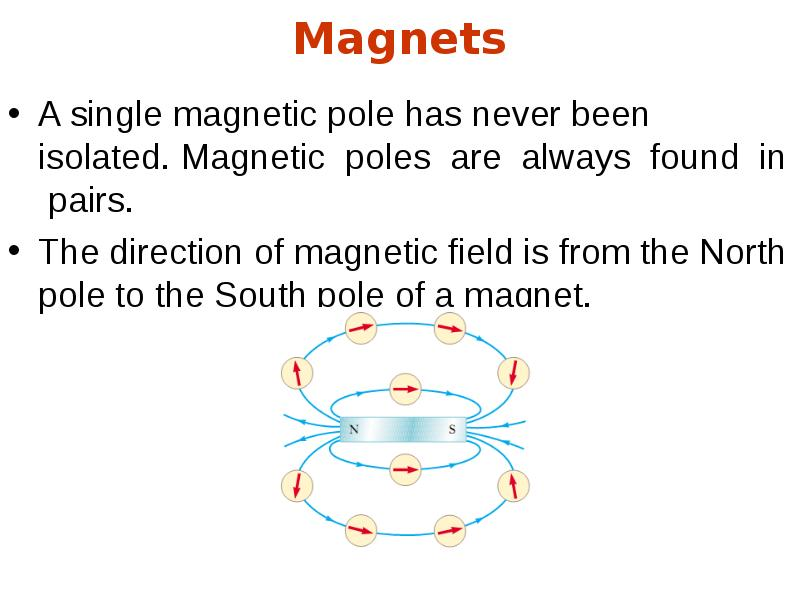Описание слайда:
Magnets A single magnetic pole has never been isolated. Magnetic poles are always found in pairs. The direction of magnetic field is from the North pole to the South pole of a magnet.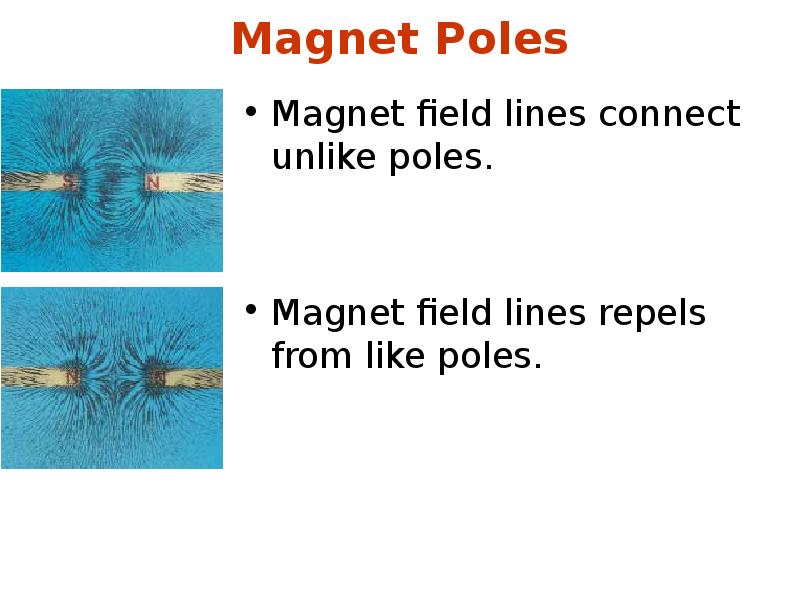Описание слайда:
Magnet Poles Magnet field lines connect unlike poles. Magnet field lines repels from like poles.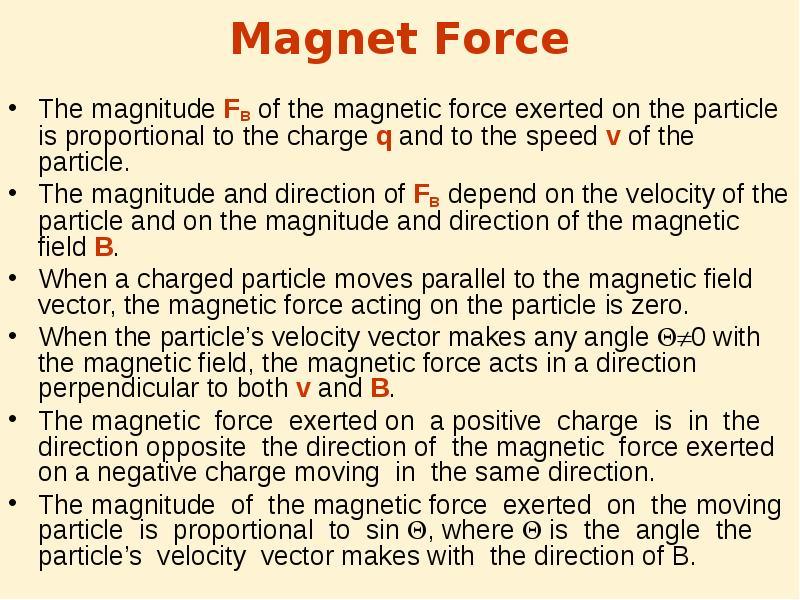Описание слайда:
Magnet Force The magnitude FB of the magnetic force exerted on the particle is proportional to the charge q and to the speed v of the particle. The magnitude and direction of FB depend on the velocity of the particle and on the magnitude and direction of the magnetic field B. When a charged particle moves parallel to the magnetic field vector, the magnetic force acting on the particle is zero. When the particle’s velocity vector makes any angle 0 with the magnetic field, the magnetic force acts in a direction perpendicular to both v and B. The magnetic force exerted on a positive charge is in the direction opposite the direction of the magnetic force exerted on a negative charge moving in the same direction. The magnitude of the magnetic force exerted on the moving particle is proportional to sin , where  is the angle the particle’s velocity vector makes with the direction of B.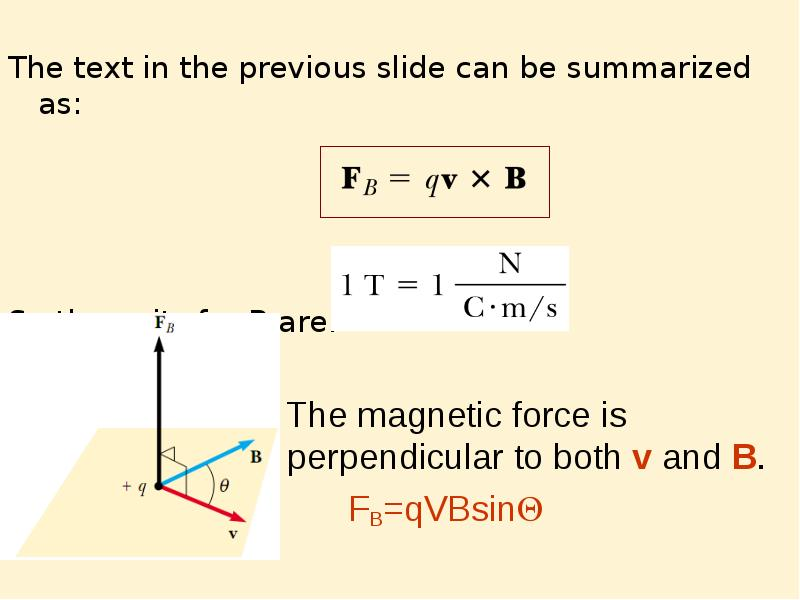Описание слайда:
The text in the previous slide can be summarized as: So the units for B are: The magnetic force is perpendicular to both v and B. FB=qVBsin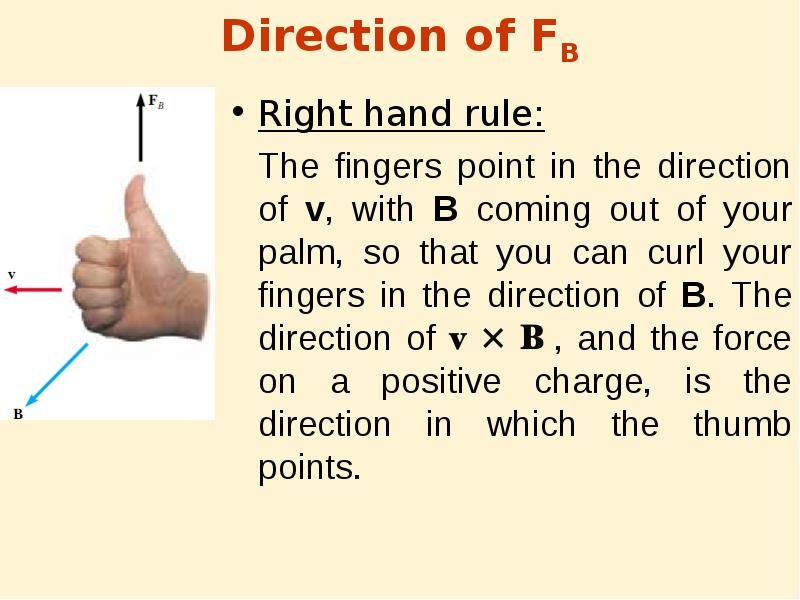Описание слайда:
Direction of FB Right hand rule: The fingers point in the direction of v, with B coming out of your palm, so that you can curl your fingers in the direction of B. The direction of , and the force on a positive charge, is the direction in which the thumb points.Описание слайда:
Magnetic field direction Magnetic field lines coming out of the paper are indicated by dots, representing the tips of arrows coming outward. Magnetic field lines going into the paper are indicated by crosses, representing the feathers of arrows going inward.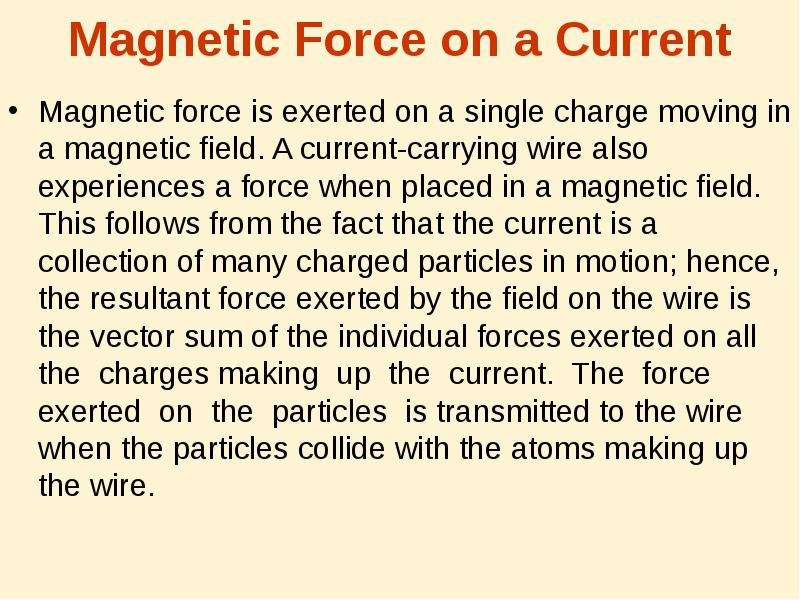Описание слайда:
Magnetic Force on a Current Magnetic force is exerted on a single charge moving in a magnetic field. A current-carrying wire also experiences a force when placed in a magnetic field. This follows from the fact that the current is a collection of many charged particles in motion; hence, the resultant force exerted by the field on the wire is the vector sum of the individual forces exerted on all the charges making up the current. The force exerted on the particles is transmitted to the wire when the particles collide with the atoms making up the wire.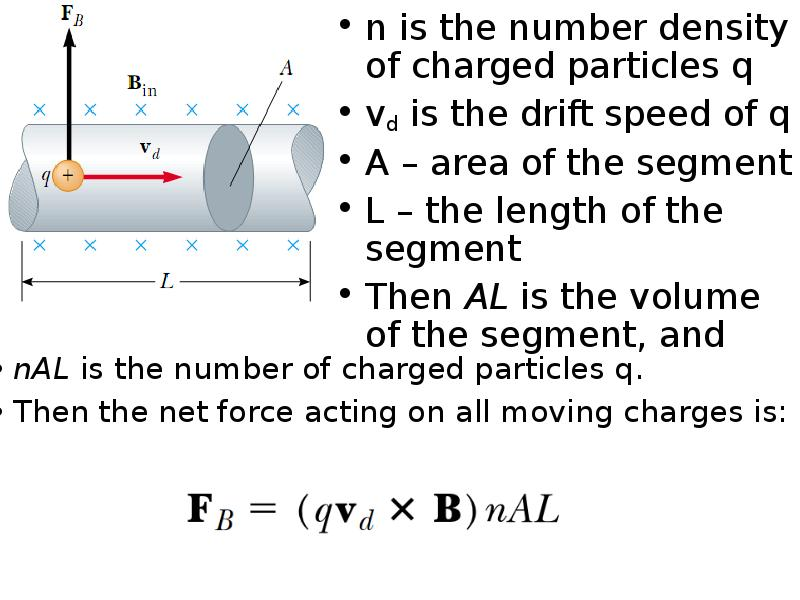Описание слайда:
n is the number density of charged particles q n is the number density of charged particles q vd is the drift speed of q A – area of the segment L – the length of the segment Then AL is the volume of the segment, and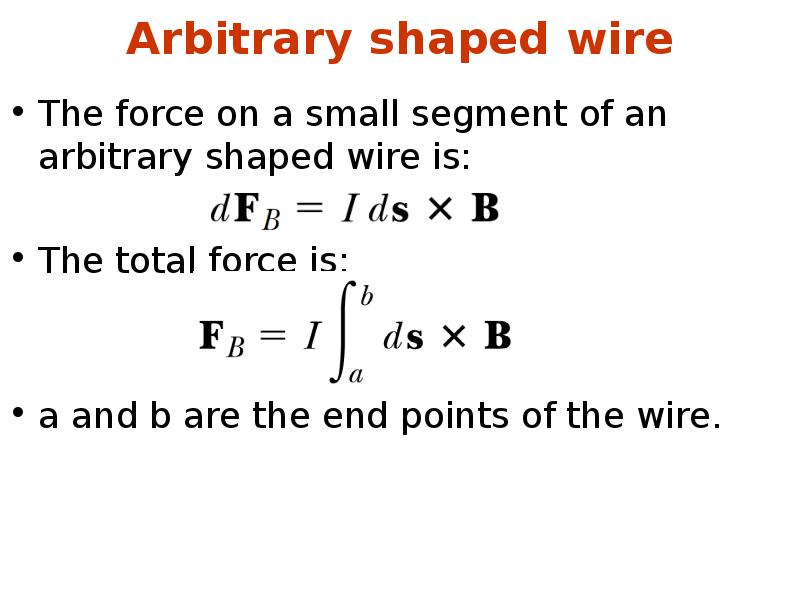Описание слайда:
Arbitrary shaped wire The force on a small segment of an arbitrary shaped wire is: The total force is: a and b are the end points of the wire.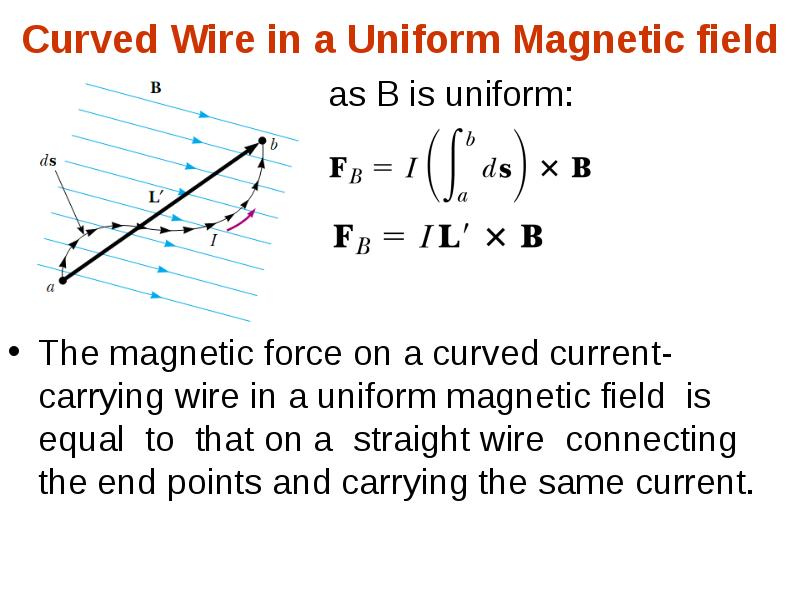Описание слайда:
Curved Wire in a Uniform Magnetic field as B is uniform: The magnetic force on a curved current-carrying wire in a uniform magnetic field is equal to that on a straight wire connecting the end points and carrying the same current.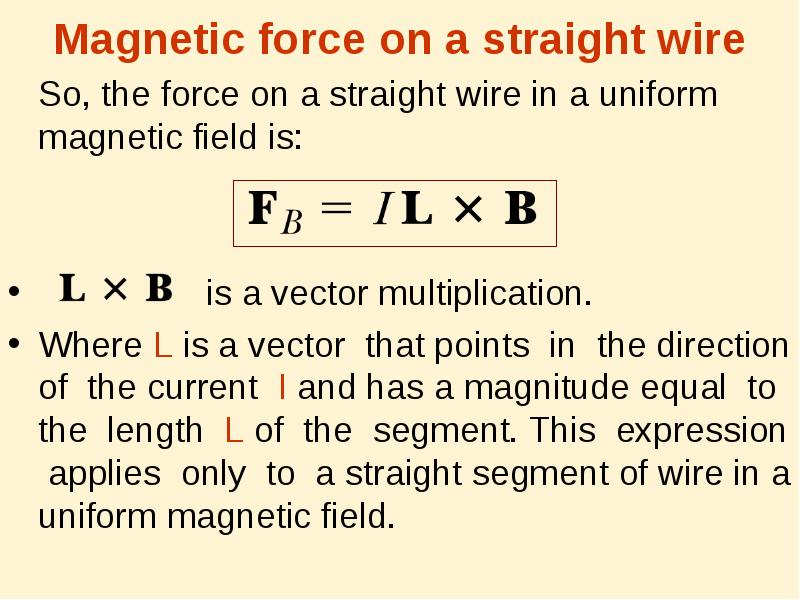Описание слайда:
Magnetic force on a straight wire So, the force on a straight wire in a uniform magnetic field is: is a vector multiplication. Where L is a vector that points in the direction of the current I and has a magnitude equal to the length L of the segment. This expression applies only to a straight segment of wire in a uniform magnetic ﬁeld.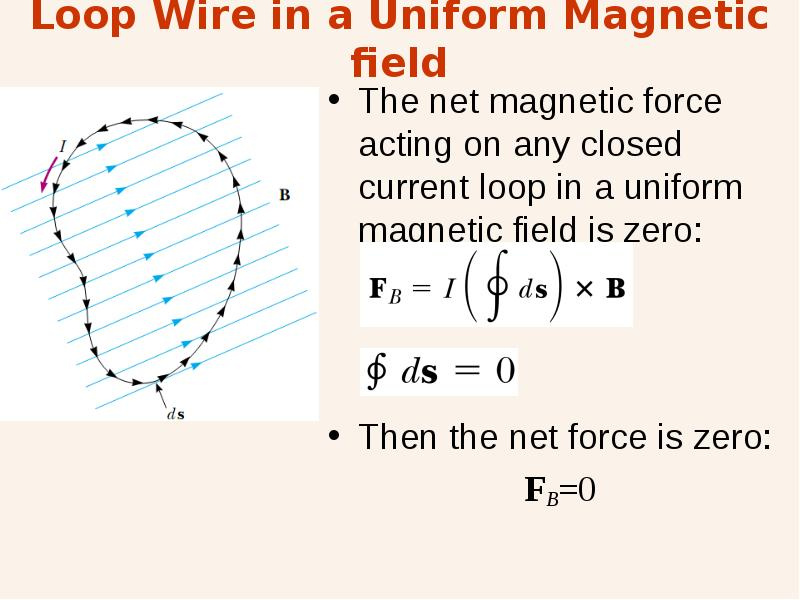Описание слайда:
Loop Wire in a Uniform Magnetic field The net magnetic force acting on any closed current loop in a uniform magnetic field is zero: Then the net force is zero: FB=0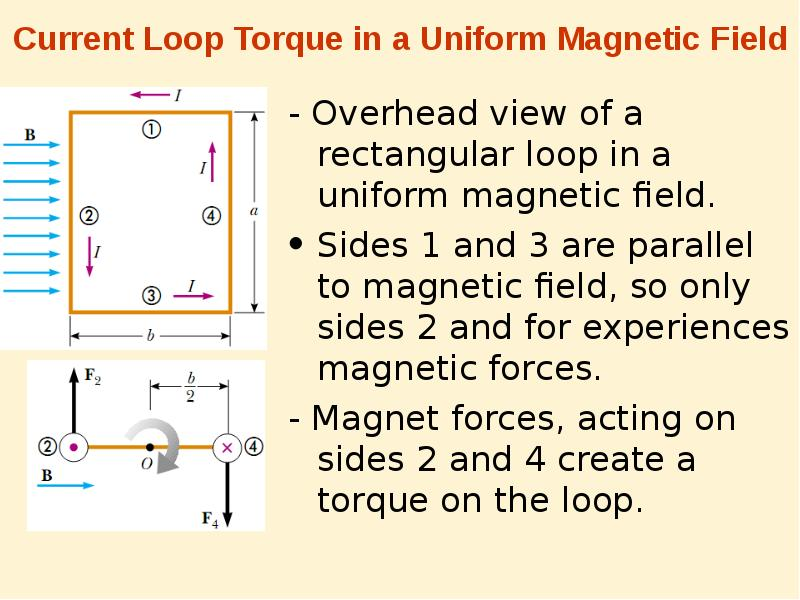Описание слайда:
Current Loop Torque in a Uniform Magnetic Field - Overhead view of a rectangular loop in a uniform magnetic field. Sides 1 and 3 are parallel to magnetic field, so only sides 2 and for experiences magnetic forces. - Magnet forces, acting on sides 2 and 4 create a torque on the loop.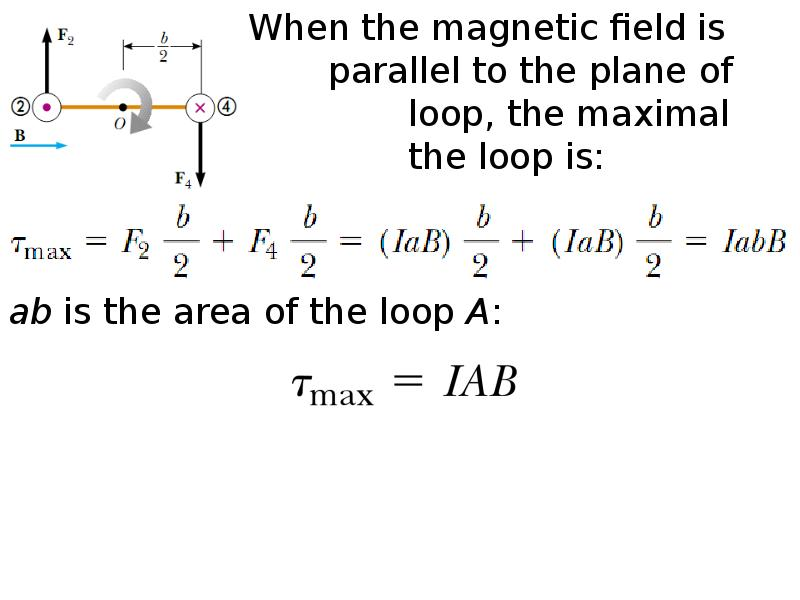Описание слайда:
When the magnetic field is parallel to the plane of the loop, the maximal torque on the loop is: When the magnetic field is parallel to the plane of the loop, the maximal torque on the loop is: ab is the area of the loop A: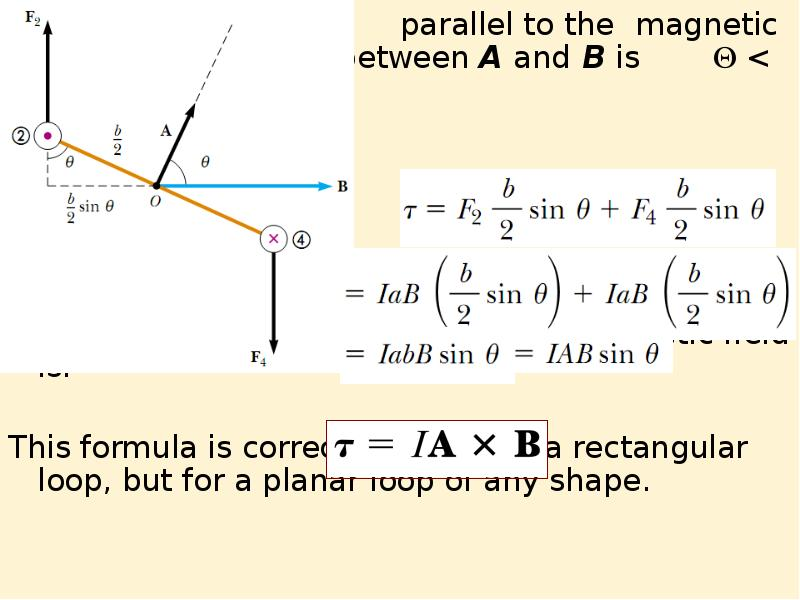Описание слайда:
When the loop is not parallel to the magnetic field, i.e. the angle between A and B is  < 90° then: When the loop is not parallel to the magnetic field, i.e. the angle between A and B is  < 90° then: So the torque on a loop in a uniform magnetic field is: This formula is correct not only for a rectangular loop, but for a planar loop of any shape.Описание слайда:
Area Vector In formula for torque we have vector A: - Its direction is perpendicular to the plane of the loop, - its magnitude is equal to the area of the loop. We determine the direction of A using the right-hand rule. When you curl the fingers of your right hand in the direction of the current in the loop, your thumb points in the direction of A.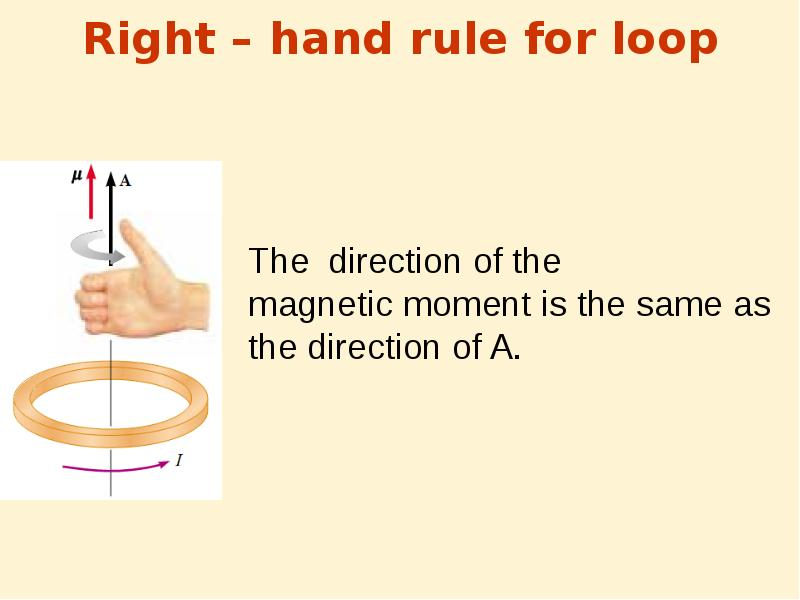Описание слайда:
Right – hand rule for loop The direction of the magnetic moment is the same as the direction of A.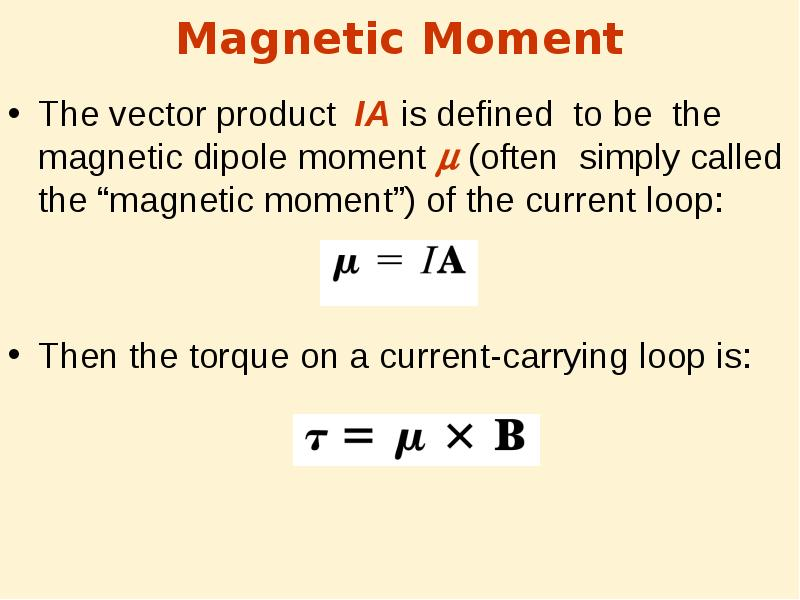Описание слайда:
Magnetic Moment The vector product IA is defined to be the magnetic dipole moment  (often simply called the “magnetic moment”) of the current loop: Then the torque on a current-carrying loop is: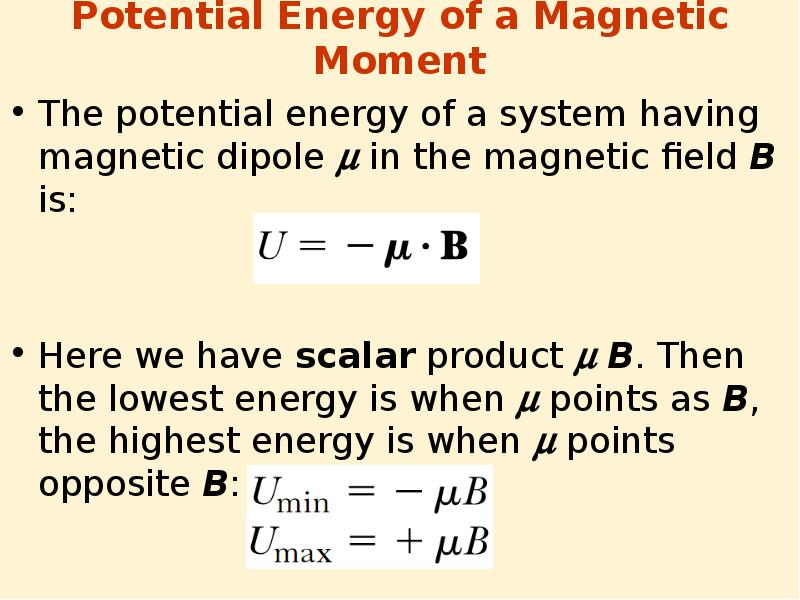Описание слайда:
Potential Energy of a Magnetic Moment The potential energy of a system having magnetic dipole  in the magnetic field B is: Here we have scalar product B. Then the lowest energy is when  points as B, the highest energy is when  points opposite B: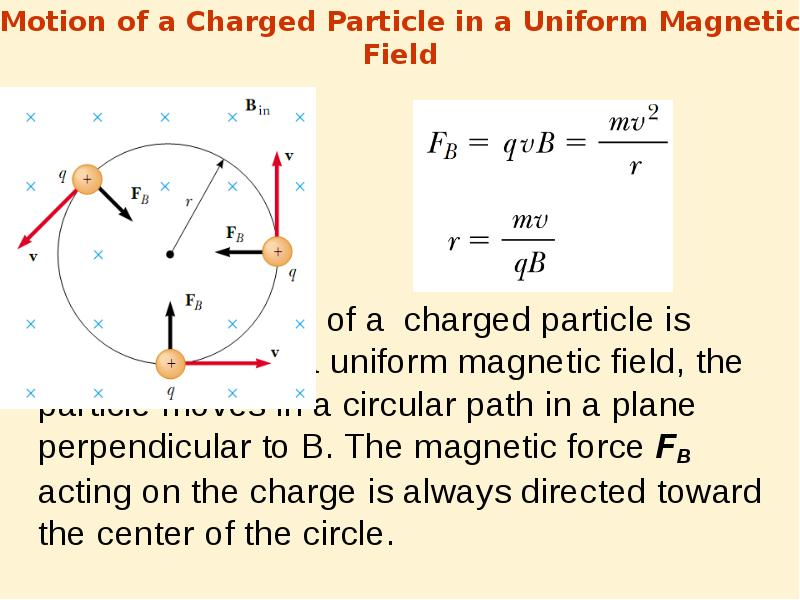Описание слайда:
Motion of a Charged Particle in a Uniform Magnetic Field When the velocity of a charged particle is perpendicular to a uniform magnetic field, the particle moves in a circular path in a plane perpendicular to B. The magnetic force FB acting on the charge is always directed toward the center of the circle.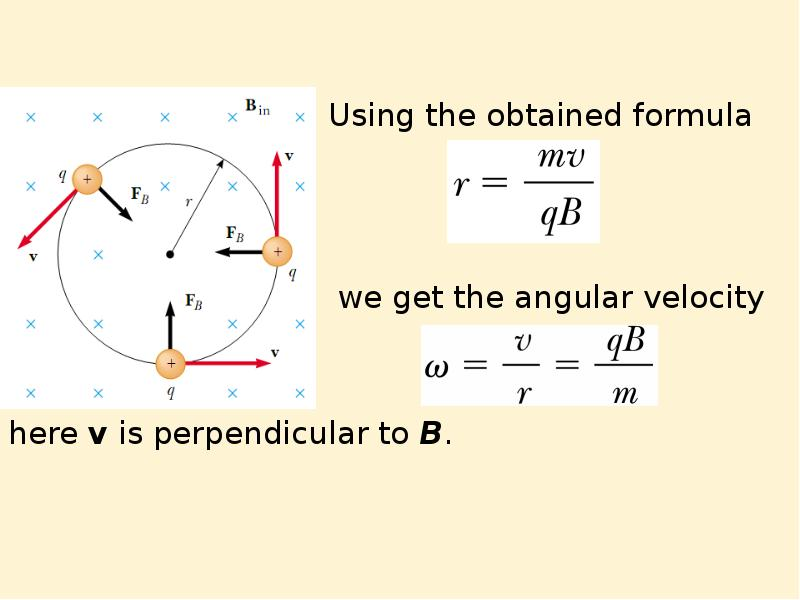Описание слайда:
Using the obtained formula we get the angular velocity here v is perpendicular to B.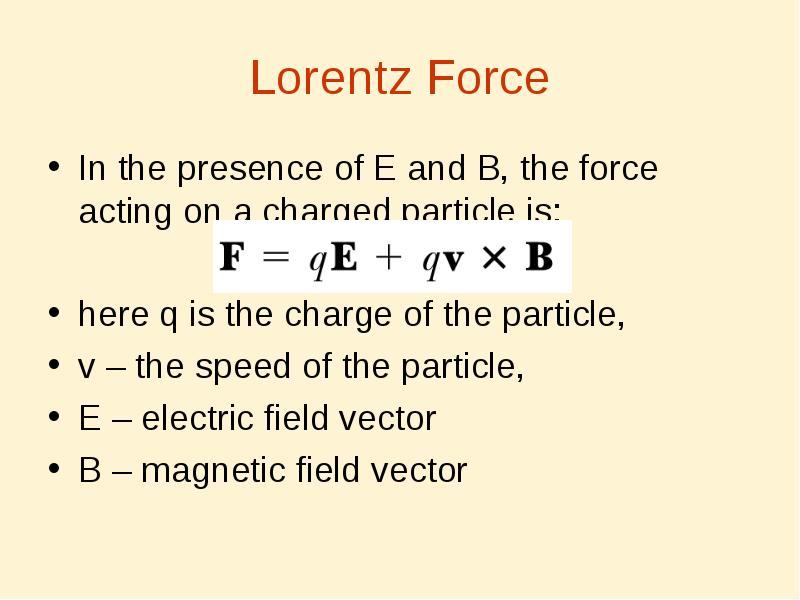Описание слайда:
Lorentz Force In the presence of E and B, the force acting on a charged particle is: here q is the charge of the particle, v – the speed of the particle, E – electric field vector B – magnetic field vector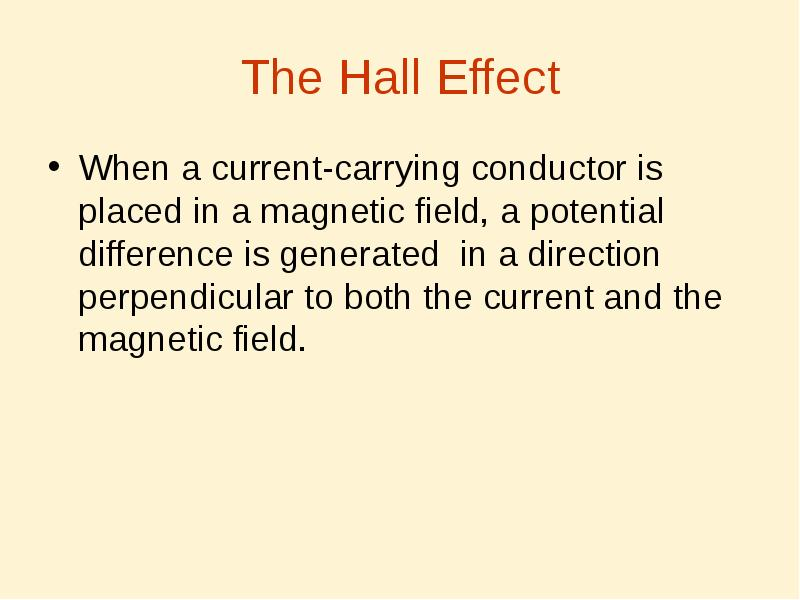Описание слайда:
The Hall Effect When a current-carrying conductor is placed in a magnetic field, a potential difference is generated in a direction perpendicular to both the current and the magnetic field.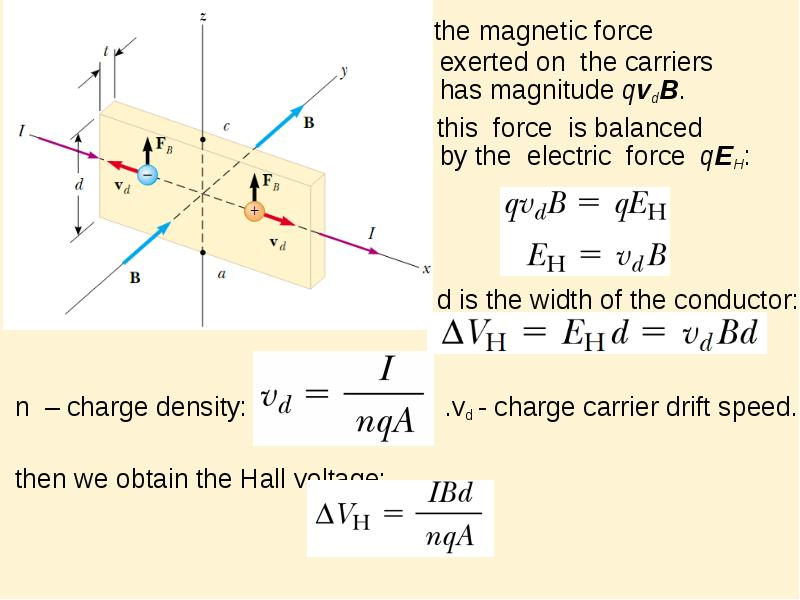Описание слайда:
the magnetic force exerted on the carriers has magnitude qvdB. the magnetic force exerted on the carriers has magnitude qvdB. this force is balanced by the electric force qEH: d is the width of the conductor: n – charge density: .vd - charge carrier drift speed. then we obtain the Hall voltage: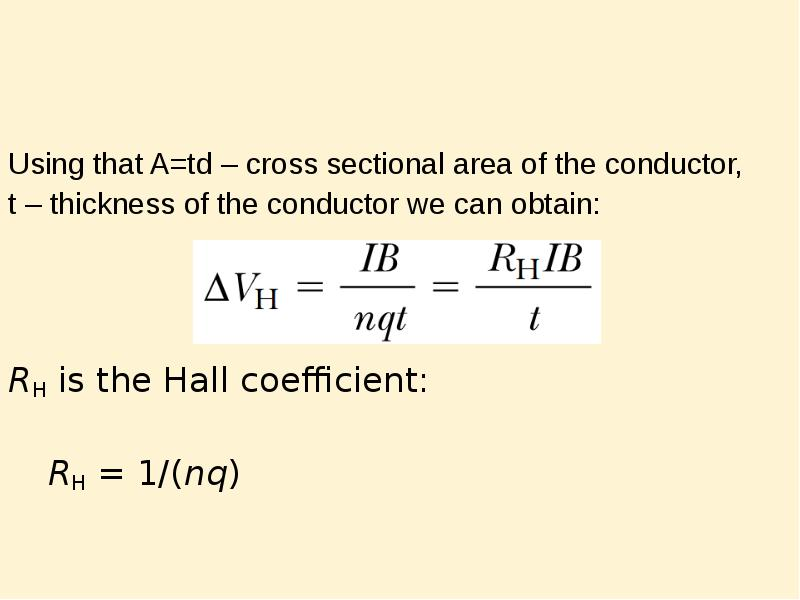Описание слайда:
Using that A=td – cross sectional area of the conductor, Using that A=td – cross sectional area of the conductor, t – thickness of the conductor we can obtain: RH is the Hall coefficient: RH = 1/(nq)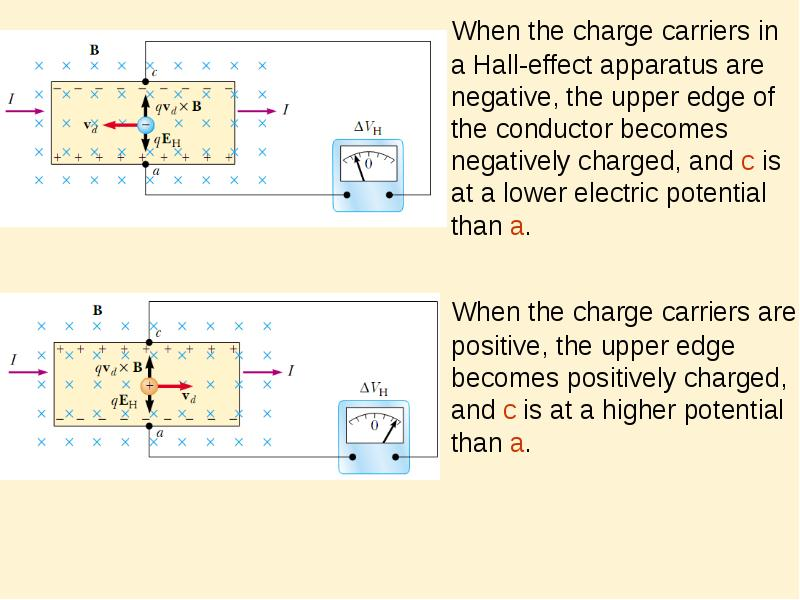Описание слайда:
When the charge carriers in a Hall-effect apparatus are negative, the upper edge of the conductor becomes negatively charged, and c is at a lower electric potential than a. When the charge carriers in a Hall-effect apparatus are negative, the upper edge of the conductor becomes negatively charged, and c is at a lower electric potential than a. When the charge carriers are positive, the upper edge becomes positively charged, and c is at a higher potential than a.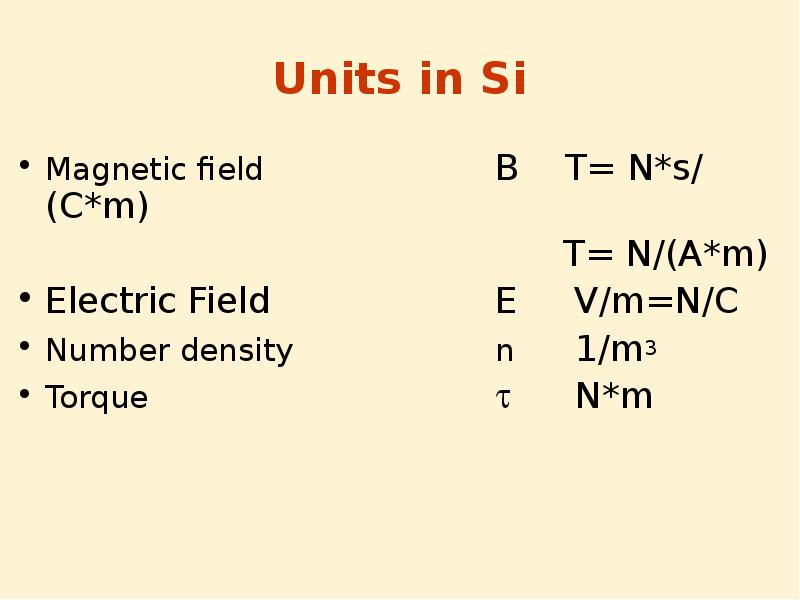Описание слайда:
Units in Si Magnetic field B T= N*s/(C*m) T= N/(A*m) Electric Field E V/m=N/C Number density n 1/m3 Torque  N*m

Скачать презентацию на тему Currents in мetals можно ниже:

Похожие презентации